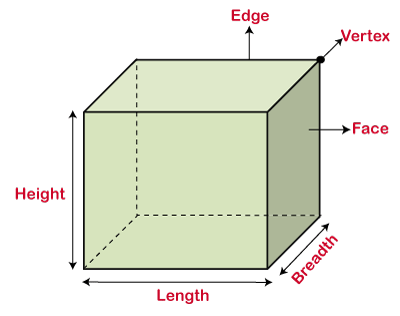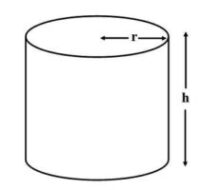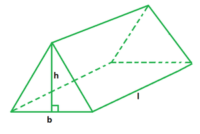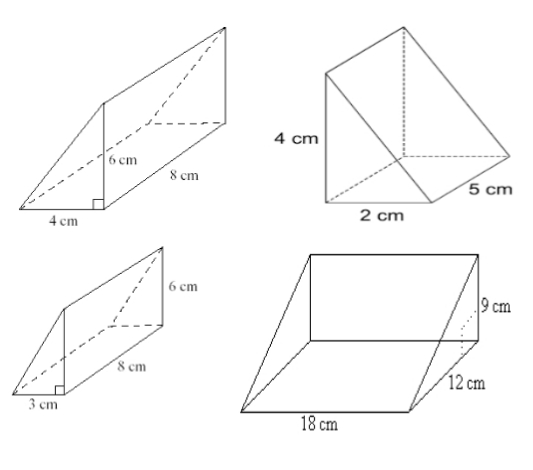# Volume and Capacity of Three Dimensional Shapes – Cube, Cuboid, Prism, Cylinder, Cone, etc. Primary 6 (Basic 6) Term 3 Week 5 Mathematics

### MATHEMATICS

THIRD TERM

WEEK 5

PRIMARY 6

THEME – MEASUREMENT

PREVIOUS LESSON – Time, Distance and Average Speed | Word Problems (Real Life Application) Primary 6 (Basic 6) Term 3 Week 4 Mathematics

### LEARNING AREA

1. Introduction

2. Volume of Cube and Cuboid

3. Volume of Cylinders and Sphere

4. Volume of Prisms

5. Lesson Evaluation and Weekly Assessment (Test)

### PERFORMANCE OBJECTIVES

By the end of the lesson, most of the pupils should have attained the following objectives –

1. identify the relationship between capacity and volume

2. convert the units of capacity.

3. solve word problems on capacity.

4. identify the three dimensional shapes such as cube, cuboid, cylinder, etc.

5. find the volume of cubes, cuboids, prisms, cylinders and spheres.

6. solve some quantitative aptitude problem on volume of prism, cylinder and sphere.

### ENTRY BEHAVIOUR

The pupils can identify the real objects of three dimensional shapes.

### INSTRUCTIONAL MATERIALS

The teacher will teach the lesson with the aid of real objects of three dimensional shapes.

### METHOD OF TEACHING

Choose a suitable and appropriate methods for the lessons.

Note – Irrespective of choosing methods of teaching, always introduce an activities that will arouse pupil’s interest or lead them to the lessons.

### REFERENCE MATERIALS

1. Scheme of Work

2. 9 – Years Basic Education Curriculum

3. Course Book

4. All Relevant Material

5. Online Information

### LESSON 1 – INTRODUCTION

ACTIVITY 1 – CAPACITY AND ITS UNITS

Capacity is the amount of solid, liquid or gas substance in a container.

The basic units of measurement of capacity are milliliter (mℓ), Centiliter (cℓ) and liter (ℓ).

The standard unit of measuring capacity is the liter (ℓ).

Small amount of liquid is measured in milliliter (mℓ) and Centiliter (cℓ) while large amount is measured in liter (ℓ).

It is equal to a thousandth of a liter, that’s, 1 ℓ = 1000 mℓ.

The relationship between milliliter (mℓ), Centiliter (cℓ) and liter (ℓ) is,

• 1 000 mℓ = 1 ℓ
• 10 cℓ = 1 mℓ
• 100 cℓ = 1 ℓ

CONVERSION OF LITRES AND MILLILITRES

Working Example 1

Convert 5 000 mℓ to litres.

1st, divide the number of millilitres by 1 000,

5 000 ÷ 1 000 = 5

2nd, change the unit to litres,

5 000 mℓ = 5 ℓ

Working Example 2

Convert 9 ℓ to millilitres.

1st, multiply the number of ℓ by 1 000,

9 x 1 000 = 9 000

2nd, change the unit of millilitres

9 ℓ = 9 000 mℓ

Working Example 3

Convert 950 cℓ to litres.

1st, divide the number of  ℓ by 100,

950/100 = 9.5

2nd, change the unit of litres,

9 cℓ = 9.5 ℓ

Working Example 2

Convert 1.5 ℓ to centilitres.

1st, multiply the number of ℓ by 100,

1.5 x 100 = 150

2nd, change the unit of centilitres,

1.5 ℓ = 150 cℓ

ACTIVITY 2 – VOLUME AND ITS VOLUME

The volume of an object is the amount of space occupied by the object.

For example, the amount of objects in a container such as cartoon, bottle or plastic containers.

The standard units of volume are in,

• Cubic metres (m³)
• Cubic centimetres (cm³)
• Cubic millimetres (mm³)

ACTIVITY 3 – DIFFERENT BETWEEN CAPACITY AND VOLUME

The difference between capacity and volume are as follows:

1. Capacity is the amount of substance in a container.

The volume of an object (container) is the amount of space inside that object (container).

2. Capacity is measured in metric units such as liters, gallons, etc.

Volume is measured in cubic units, such as cubic centimeter, cubic meter.

3. Capacity, only hollow objects have capacity.

Volume, both solid and hollow objects have volume.

### LESSON 2 – VOLUME OF THREE DIMENSIONAL SHAPES

ACTIVITY 1 – INTRODUCTION (REVISION)

Three dimensional shapes (3D) are objects or shapes that three dimensions such as length, width, and height.

Examples of three dimensional shapes are cube, cuboid, triangular prism, sphere, cone, cylinder and pyramid.

The properties of a three dimensional shapes are faces, edges and vertices.

The volume of an object is the amount of space occupied by the object and it can be easily calculated by using arithmetic formulas.

ACTIVITY 2 – VOLUME OF A CUBE

A cube is a solid three dimensional shape which has 6 square faces.

Examples of cube are ice cube, cube square, dice, etc.

The cube has the following properties, 8 vertices (corners), 12 edges and 6 faces.The volume of a cube is Length × Breadth × Height.Since, L = B = H

Therefore, the volume of a cube is a³ depending on the name given to the sides.

That’s,

### V = a³ cube units

For example, find the volume of a cube whose length is 5 cm.

V = L x B x H or a³

= 5 x 5 x 5 or 5³

= 125 cm³

ACTIVITY 3 – VOLUME OF A CUBOID

A cuboid is a solid three dimensional shape which has 6 rectangular faces or 4 rectangular faces and 2 square faces.

Examples of cuboid are the lunch box, bricks, books, carton, cupboard, fridge, etc.

It is also called a rectangular prism.

The faces of the cuboid are a rectangle in shape.

The cuboid has the following properties, 8 vertices (corners), 12 edges and 6 faces.

The volume of a cube is Length × Breadth × Height.That’s,

### V = L x B x H cube units

For example,

Find the volume of a cuboid whose length 7 cm, breadth = 5 cm and height = 3 cm.

V = L x B x H, where L = 7 cm, B = 5 cm and 3 cm.

= 7 x 5 x 3

= 105 cm³

Note – Teacher guides pupils to,

• identify the length, breadth and height of cube and height.
• differentiate between the lengths of cube and cuboid.

ACTIVITY 3 – WORKING EXERCISE (HOME WORK)

Fill in the blanks,

1. The volume of a cube is v = ________.

2. The volume of a cuboid v = ________ × ________ × ________.

3. The volume of a 5 cm cube is v = ________.

Find the volume of the cuboid whose dimensions are,

4. Length = 7 m, Breadth = 6 m, Height = 4 m.

5. Length = 14 cm, Breadth = 14 cm, Height = 26 cm.

6. Length = 24 m, Breadth = 4 m, Height = 5 m.

7. A cuboid measuring 5 cm × 7 cm × 6 cm and a cube of 5cm are kept on a table. Which shape has less volume?

8. A cuboid is 8 cm long, 4 cm width and 9 cm high and a cube is 5 cm long. Which one has more volume?

9. What is the volume of an indomine cartoon with length 15 cm, breadth 25 cm and height 12 cm?

10. A box is 15 cm in length, 8 cm in breadth and 5 cm in height. How many box will be used to pack a good of length 15 cm, breadth 10 cm and height 8 cm?

11. A tank is 50 m long, 30 m wide and 2 m deep. Find the capacity of the tank in cubic metre.

12. Treasurer’s box is 60 cm long, 30 cm wide and 70 cm high. What is the capacity of the box?

13. Find the volume of the following cube and cuboid shapes,### LESSON 3 – VOLUME OF CYLINDER AND SPHERE

ACTIVITY 1 – VOLUME OF A CYLINDER

A cylinder is defined as a three dimensional shapes with 2 circular bases connected by a curved surface.

Examples of cylinder pipes, cans, tanks, battery, gas cylinder, fire extinguisher, etc.

The cylinder has the following properties, zero vertex, 2 edges, 2 flat faces – circles and one curved face

The volume of the cylinder is the area of base multiply by the height.V = A x h, since the area of a circle = πr².

Therefore,

### V = πr²h cubic units, where π = 22/7 or 3.1423

For example,

1. Calculate the volume of a cylinder whose height 18 cm and base radius of 16 cm. (Take π = 22/7).

V = πr²h, where π = 22/7, r = 7 cm and h = 18 cm

V = 22/7 x 7² x 18 or 22/7 x 7 x 7 x 18

= 19404/7

= 2 772 cm³

2. A cylindrical tin of milk has its base diameter as 14 cm and height as 10 cm. Calculate the volume of tomato puree that can be contained in it (Take π = 22/7).

Volume = π r²h where, π = 22/7, r = 14/2 = 7 cm and h = 10 cm.

V = 22/7 ¹ x 7 ¹ cm x 7 cm x 10 cm.

= 22 x 1 cm x 7 cm x 10 cm

= 1, 540 cm².

ACTIVITY 2 – VOLUME OF A SPHERE

A sphere is a three dimensional round shaped object with just one face.

Examples of sphere ball, moon, sun, eyeball, orange, etc.

The sphere has the following properties, no vertex, no edge and one curved face.

The volume of sphere is the amount of air that a sphere can be held inside it.That’s,

### V = 4/3 πr³ cube units

For example,

1. Find the volume of a sphere whose radius is 6 cm?

V = 4/3 πr³, where π = 22/7 and r = 6 cm.

= 4/7 x 22/7 x 6³

= (4 X 22 x 216) / (7 x 7)

= 19008/49

= 387.91 cm³

2. A solid sphere has a radius of 2 cm. Find the volume. Take π = 22/7

V = 4/3 π r³

= 4/3 x 22/7 x 2 cm x 2 cm x 2 cm

= 88/21 x 8 cm³

= 704/21 cm³

= 35.5 cm³.

ACTIVITY 3 – WORKING EXERCISE (HOME WORK)

Fill in the blanks,

1. The volume of a cylinder is v = ________ × ________ × ________.

2. The volume of a sphere v = ________ × ________ × ________.

3. Find the volume of a cylinder with a circular side whose the radius is 7 cm and length is 12 cm.

4. Calculate the volume of a cylinder whose height is 12 cm, and a base with a radius is 14 cm.

5. A cylinder has a radius of r = 6 cm and a height of h = 54 cm. What is its volume?

6. Find the volume of a sphere with a radius of 24 cm.

7. What is the volume of a sphere whose radius is diameter is 16 cm?

8. What is the volume of a sphere with a radius of 21 m?

9. What is the volume of a sphere with a diameter of 12 cm long?

10. Find the sum of the volumes whose radius is 6 cm and diameter is 18 cm.

### LESSON 4 – VOLUME OF TRIANGULAR PRISMS

ACTIVITY 1 – INTRODUCTION

A triangular prism is a solid three dimensional shape which has 5 faces (2 triangles and 3 rectangles).

Examples of triangular prism are triangular roofs, camping tents, and chocolate candy bars.

The triangular prism has the following properties, 6 vertices, 9 edges and faces.

The volume of a triangular prism is Base area × Length.Where, base area = area of the base triangle and length = length of the triangular prism (also known as the height of the prism)

That’s,

### V = ½ B x H x L or ½ x L x B x H cube units

For example, find out the volume of a triangular prism whose base are is 6 cm, length is 4 cm and height is 8 cm.

V = ½ B x H x L, where B = 6 cm, H = 8 cm and L = 4 cm

= ½ x 6 x 8 x 4

= 192/2

= 96 cm³

ACTIVITY 2 – VOLUME OF PYRAMID

A pyramid is also a three dimensional shape with a square base and four triangular faces.

Examples of pyramid are roof of a building, Egyptian pyramid, groundnut pyramid, etc.

There are different types of pyramid depending on their based.

We have the triangular, square, quadrilateral base but the most commonly used is the square pyramid.

The pyramid has the following properties, 5 vertices, 8 edges and 5 faces.

The volume V of a pyramid is one – third the area of the base times the height.

V = 1/3 B x H, where base area is L x B.

That’s,

### V = 1/3 x L x B x H or 1/3 x B x H

For example,

Find the volume of a regular square pyramid whole base sides is 10 cm and height 21 cm.

V = 1/3 x B x H, where B = 10 cm and H = 21 cm

= 1/3 x 10 x 10 x 21

= 2100/3

= 700 cm³.

2. What is the volume of a regular square pyramid whole base area is 64 cm² and height 21 cm.

V = 1/3 x B x H, where Base area is 64 cm² and Height = 21 cm.

= 1/3 x 64 x 21

= 64 x 7

= 448 cm³

ACTIVITY 3 – WORKING EXERCISE (HOME WORK)

Fill in the blanks,

1. The volume of a triangular prism is v = ½ x ________ × ________ × ________.

2. The volume of a pyramid is v = 1/3 x ________ × ________ × ________.

3. Find the volume of a pyramid with a height of 16 cm and a square base with a side length of 5 cm.

4. What is the sum of the number of vertices, edges, and faces of a square pyramid and triangular prism?

5. What is the volume of a pyramid with a height of 16 cm and a base area of 25 cm²?

6. Find the volume of the following sphere shapes,LESSON 5 – REVISION AND WEEKLY ASSESSMENT (TEST)

As stated in the working exercises.

### PRESENTATION

To deliver the lesson, the teacher adopts the following steps:

1. To introduce the lesson, the teacher revises the previous lesson. Based on this, he/she asks the pupils some questions;

2. Teacher organizes pupils in groups or pairs depending on the size of the class and available instructional materials.

3. Teacher displays different containers for pupils to identify and state their actual use and other uses.

Pupil’s Activities – State uses of different containers.

4. Teacher asks pupils to differentiate between the sizes of the containers.

5. Teacher listen to the pupils and guides them to identify the size of the each container as indicated on them. For example, 75cl, 1 litre, etc.

Pupil’s Activities – Identify the size of the each container.

6. Teacher uses the pupil’s reasonable responses to introduce and leads a discussion on capacity and volume of three dimensional shapes.

Pupil’s Activities – Pay attention to the lesson introduction to understand the concept of the lesson.

7. Teacher guides to convert the units of capacity and volume.

Pupil’s Activities – Convert one unit to another.

8. Teacher displays samples or chart should three dimensional shapes.

9. Based on the previous lesson or knowledge, teacher guides pupils as groups to identify and describe the shapes.

Pupil’s Activities – Identify the real objects of the three dimensional shapes.

10. Teacher guides pupils to identify the properties of each three different shape and to find their volume.

Pupil’s Activities – Find the volume of three dimensional shapes.

11. Teacher summarizes the lessons on the board with appropriate lesson evaluation.

Pupil’s Activities – Participate actively in the summary of the lesson by responding correctly to the questions and write as instructed.

### CONCLUSION

To conclude the lesson for the week, the teacher revises the entire lesson and links it to the following week’s lesson.

### LESSON EVALUATION

As stated in the stated.